# ML Aggarwal Class 7 Solutions for ICSE Maths Chapter 3 Rational Numbers Ex 3.1

## ML Aggarwal Class 7 Solutions for ICSE Maths Chapter 3 Rational Numbers Ex 3.1

Question 1.
Which of the following are positive rational numbers?Solution:Question 2.
Which of the following are negative rational numbers?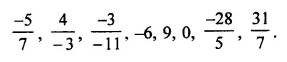Solution: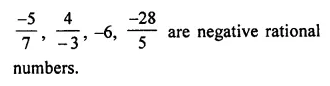Question 3.
Find four rational numbers equivalent to each of the following rational numbers:
(i) $$\frac { 3 }{ -7 }$$
(ii) $$\frac { -5 }{ -9 }$$
Solution: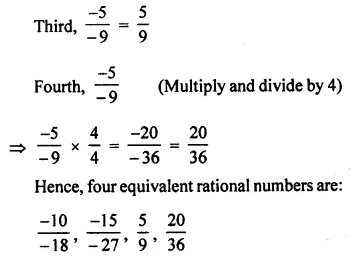Question 4.
Write each of the following ratinoal numbers with positive denominators:
(i) $$\frac { 4 }{ -9 }$$
(ii) $$\frac { 17 }{ -33 }$$
(iii) $$\frac { -15 }{ -38 }$$
Solution: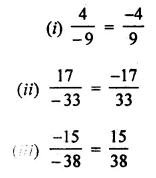Question 5.
Write next four rational numbers in each of the following patterns: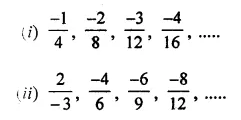Solution:
Next four rational numbers in the same patterns.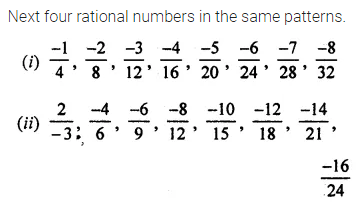Question 6.
Which of the following pairs of rational numbers are equal?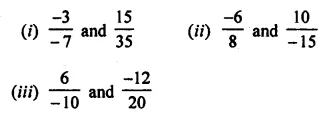Solution: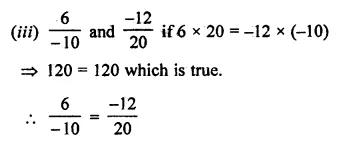Question 7.
Which of the following pairs represent the same rational number?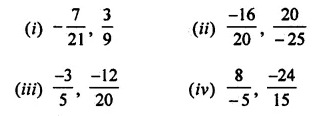Solution: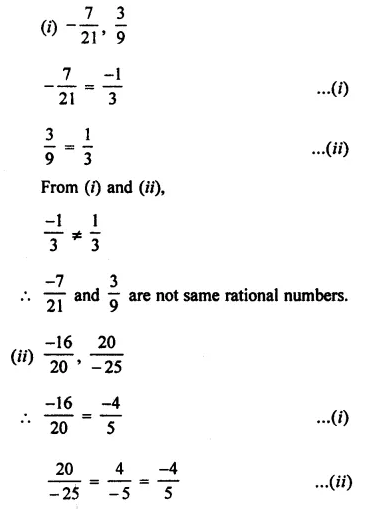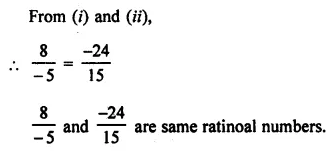Question 8.
Fill in the blanks: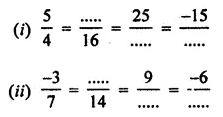Solution: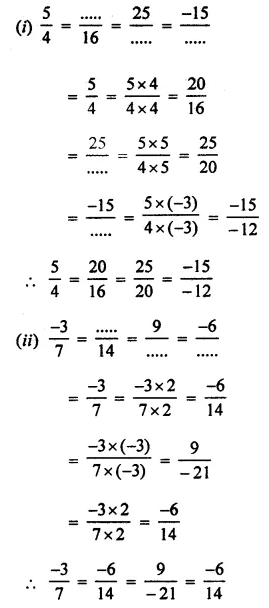Question 9.
Reduce each of the following rational numbers in standard form:Solution: